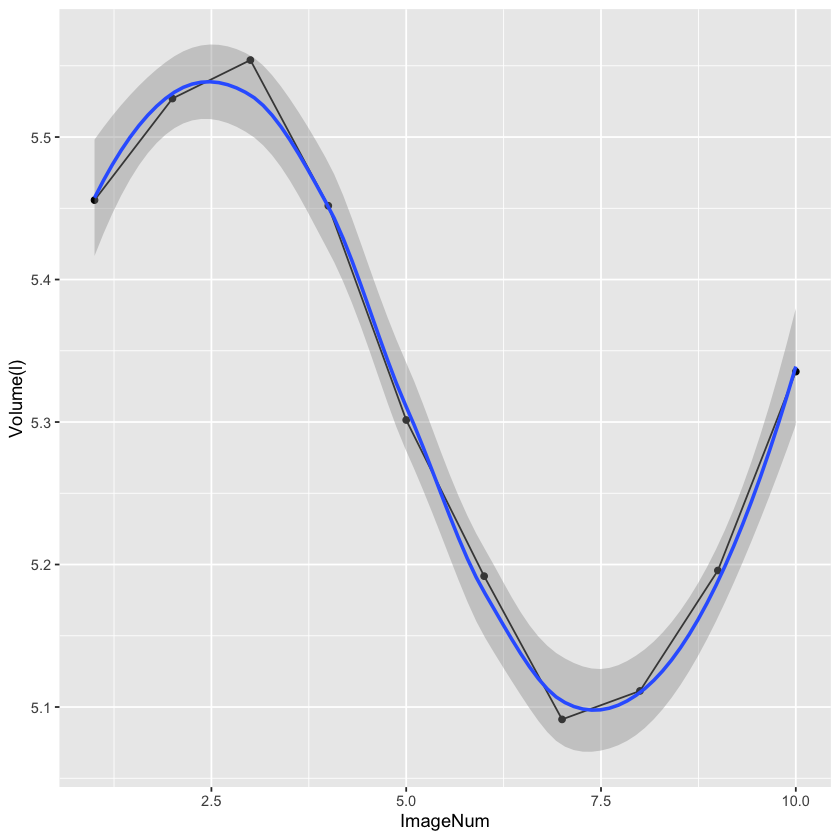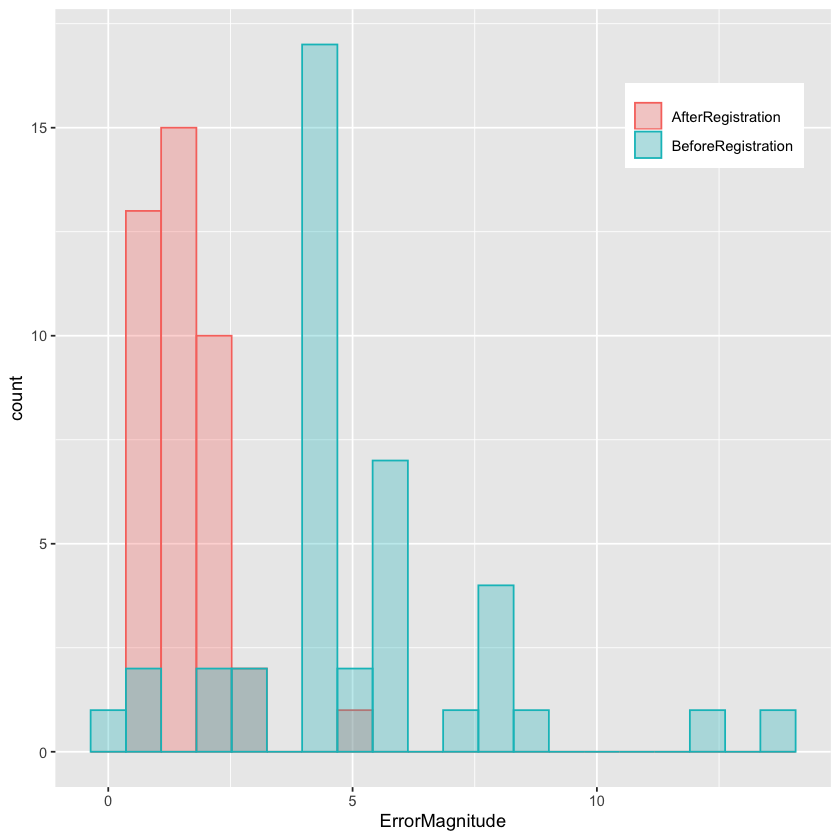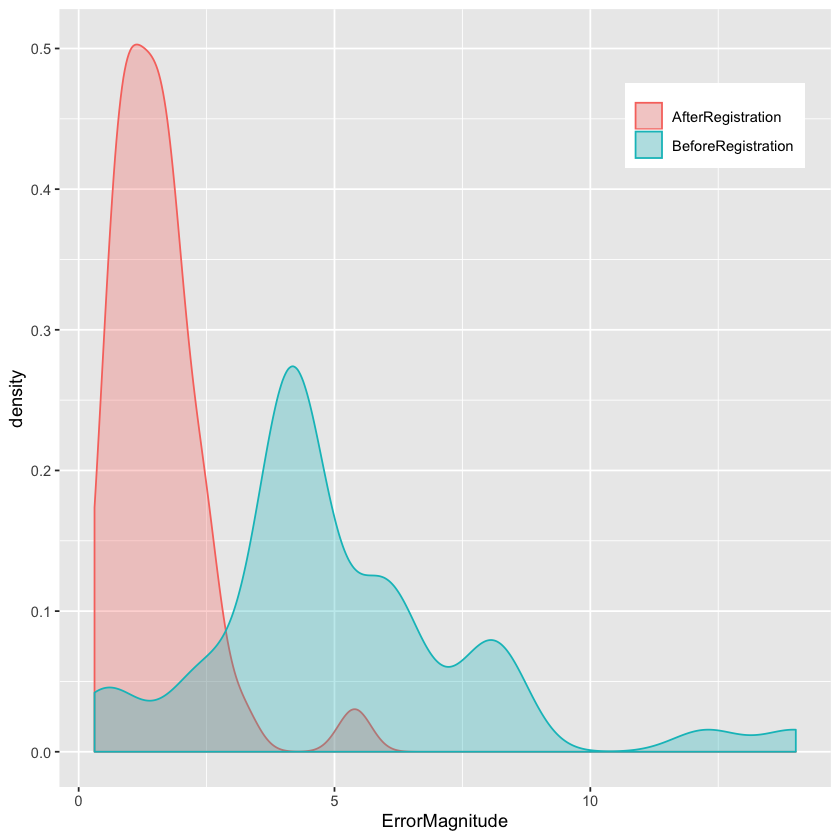# Non-Rigid Registration: Free Form Deformation

This notebook illustrates the use of the Free Form Deformation (FFD) based non-rigid registration algorithm in SimpleITK.

The data we work with is a 4D (3D+time) thoracic-abdominal CT, the Point-validated Pixel-based Breathing Thorax Model (POPI) model. This data consists of a set of temporal CT volumes, a set of masks segmenting each of the CTs to air/body/lung, and a set of corresponding points across the CT volumes.

The POPI model is provided by the Léon Bérard Cancer Center & CREATIS Laboratory, Lyon, France. The relevant publication is:

J. Vandemeulebroucke, D. Sarrut, P. Clarysse, "The POPI-model, a point-validated pixel-based breathing thorax model", Proc. XVth International Conference on the Use of Computers in Radiation Therapy (ICCR), Toronto, Canada, 2007.

The POPI data, and additional 4D CT data sets with reference points are available from the CREATIS Laboratory here.

In :
library(SimpleITK)
library(ggplot2)
library(tidyr)

# Utility method that either downloads data from the Girder repository or

Loading required package: rPython



## Utilities¶

Utility methods used in the notebook for display and registration evaluation.

In :
source("registration_utilities.R")


Load all of the images, masks and point data into corresponding lists. If the data is not available locally it will be downloaded from the original remote repository.

Take a look at a temporal slice for a specific coronal index (center of volume). According to the documentation on the POPI site, volume number one corresponds to end inspiration (maximal air volume).

You can modify the coronal index to look at other temporal slices.

In :
body_label <- 0
air_label <- 1
lung_label <- 2

image_file_names <- file.path("POPI", "meta", paste0(0:9, "0-P.mhd"))
# Read the CT images as 32bit float, the pixel type required for registration.
image_list <- lapply(image_file_names, function(image_file_name) ReadImage(fetch_data(image_file_name), "sitkFloat32"))

points_file_names <- file.path("POPI", "landmarks", paste0(0:9, "0-Landmarks.pts"))

# Look at a temporal slice for the specific coronal index
coronal_index <- as.integer(round(image_list[]$GetHeight()/2.0)) temporal_slice <- temporal_coronal_with_overlay(coronal_index, image_list, mask_list, lung_label, -1024, 976) # Flip the image so that it corresponds to the standard radiological display. Show(temporal_slice[,seq(temporal_slice$GetHeight(),0,-1),])


### Getting to know your data¶

While the POPI site states that image number 1 is end inspiration, and visual inspection seems to suggest this is correct, we should probably take a look at the lung volumes to ensure that what we expect is indeed what is happening.

Which image is end inspiration and which end expiration?

In :
volume_in_liters <- function(mask, label)
{
label_shape_statistics_filter <- LabelShapeStatisticsImageFilter()
label_shape_statistics_filter$Execute(mask) # 1mm^3 = 1e-6 liter return (0.000001*label_shape_statistics_filter$GetPhysicalSize(label))
}

# plot the original data and a smoothed version
ggplot(lungdf, aes(x=ImageNum, y=Volume)) + geom_point() + geom_line() + ylab("Volume(l)") +
geom_smooth(method='loess')## Free Form Deformation¶

This function will align the fixed and moving images using a FFD. If given a mask, the similarity metric will be evaluated using points sampled inside the mask. If given fixed and moving points the similarity metric value and the target registration errors will be displayed during registration.

As this notebook performs intra-modal registration, we use the MeanSquares similarity metric (simple to compute and appropriate for the task).

In :
bspline_intra_modal_registration <- function(fixed_image, moving_image, fixed_image_mask=NULL)
{
registration_method <- ImageRegistrationMethod()

# Determine the number of Bspline control points using the physical spacing we want for the control grid.
grid_physical_spacing <- c(50.0, 50.0, 50.0) # A control point every 50mm
image_physical_size <- fixed_image$GetSize() * fixed_image$GetSpacing()
mesh_size <- as.integer(round(image_physical_size/grid_physical_spacing))

initial_transform <- BSplineTransformInitializer(image1 = fixed_image,
transformDomainMeshSize = mesh_size, order=3)
registration_method$SetInitialTransform(initial_transform) registration_method$SetMetricAsMeanSquares()
# Settings for metric sampling, usage of a mask is optional. When given a mask the sample points will be
# generated inside that region. Also, this implicitly speeds things up as the mask is smaller than the
# whole image.
registration_method$SetMetricSamplingStrategy("RANDOM") registration_method$SetMetricSamplingPercentage(0.01)
{
registration_method$SetMetricFixedMask(fixed_image_mask) } # Multi-resolution framework. registration_method$SetShrinkFactorsPerLevel(shrinkFactors = c(4,2,1))
registration_method$SetSmoothingSigmasPerLevel(smoothingSigmas=c(2,1,0)) registration_method$SmoothingSigmasAreSpecifiedInPhysicalUnitsOn()

registration_method$SetInterpolator("sitkLinear") registration_method$SetOptimizerAsLBFGSB(gradientConvergenceTolerance=1e-5, numberOfIterations=100)

return(registration_methodExecute(fixed_image, moving_image)) }  ## Perform Registration¶ The following cell allows you to select the images used for registration, runs the registration, and afterwards computes statistics comparing the target registration errors before and after registration and displays a histogram of the TREs. In : # Select the fixed and moving images, valid entries are in [1,10]. fixed_image_index <- 1 moving_image_index <- 8 tx <- bspline_intra_modal_registration(fixed_image = image_list[[fixed_image_index]], moving_image = image_list[[moving_image_index]], fixed_image_mask = (mask_list[[fixed_image_index]] == lung_label)) initial_errors <- registration_errors(Euler3DTransform(), points_list[[fixed_image_index]], points_list[[moving_image_index]]) final_errors <- registration_errors(tx, points_list[[fixed_image_index]], points_list[[moving_image_index]]) df <- data.frame(AfterRegistration=final_errors, BeforeRegistration=initial_errors) df.long <- gather(df, key=ErrorType, value=ErrorMagnitude) ggplot(df.long, aes(x=ErrorMagnitude, group=ErrorType, colour=ErrorType, fill=ErrorType)) + geom_histogram(bins=20,position='identity', alpha=0.3) + theme(legend.title=element_blank(), legend.position=c(.85, .85)) ## Or, if you prefer density plots ggplot(df.long, aes(x=ErrorMagnitude, group=ErrorType, colour=ErrorType, fill=ErrorType)) + geom_density(position='identity', alpha=0.3) + theme(legend.title=element_blank(), legend.position=c(.85, .85)) cat(paste0('Initial alignment errors in millimeters, mean(std): ', sprintf('%.2f',mean(initial_errors)),'(',sprintf('%.2f',sd(initial_errors)),') max:', sprintf('%.2f\n',max(initial_errors)))) cat(paste0('Final alignment errors in millimeters, mean(std): ', sprintf('%.2f',mean(final_errors)),'(',sprintf('%.2f',sd(final_errors)),') max:', sprintf('%.2f\n',max(final_errors))))Initial alignment errors in millimeters, mean(std): 5.07(2.70) max:14.02 Final alignment errors in millimeters, mean(std): 1.54(0.89) max:5.39Another option for evaluating the registration is to use segmentation. In this case, we transfer the segmentation from one image to the other and compare the overlaps, both visually, and quantitatively. Note: A more detailed version of the approach described here can be found in the Segmentation Evaluation notebook. In : # Transfer the segmentation via the estimated transformation. Use Nearest Neighbor interpolation to retain the labels. transformed_labels <- Resample(mask_list[[moving_image_index]], image_list[[fixed_image_index]], tx, "sitkNearestNeighbor", 0.0, mask_list[[moving_image_index]]GetPixelID())


In :
# Look at the segmentation overlay before and after registration for a specific coronal slice
coronal_index_registration_evaluation <- as.integer(round(image_list[[fixed_image_index]]$GetHeight()/2.0)) temporal_slice <- temporal_coronal_with_overlay(coronal_index_registration_evaluation, list(image_list[[fixed_image_index]], image_list[[fixed_image_index]]), segmentations_before_and_after, lung_label, -1024, 976) # Flip the image so that it corresponds to the standard radiological display. Show(temporal_slice[,seq(temporal_slice$GetHeight(),0,-1),])

# Compute the Dice coefficient and Hausdorff distance between the segmentations before, and after registration.
after_registration <- transformed_labels == lung_label

label_overlap_measures_filter <- LabelOverlapMeasuresImageFilter()

label_overlap_measures_filter$Execute(ground_truth, before_registration) cat(paste0('Dice coefficient before registration: ', sprintf("%.2f\n", label_overlap_measures_filter$GetDiceCoefficient())))

label_overlap_measures_filter$Execute(ground_truth, after_registration) cat(paste0('Dice coefficient after registration: ', sprintf("%.2f\n", label_overlap_measures_filter$GetDiceCoefficient())))

hausdorff_distance_image_filter <- HausdorffDistanceImageFilter()

hausdorff_distance_image_filter$Execute(ground_truth, before_registration) cat(paste0('Hausdorff distance before registration: ', sprintf("%.2f\n", hausdorff_distance_image_filter$GetHausdorffDistance())))

hausdorff_distance_image_filter$Execute(ground_truth, after_registration) cat(paste0('Hausdorff distance after registration: ', sprintf("%.2f\n", hausdorff_distance_image_filter$GetHausdorffDistance())))

Dice coefficient before registration: 0.94
Dice coefficient after registration: 0.97
Hausdorff distance before registration: 18.04
Hausdorff distance after registration: 12.31

In [ ]: